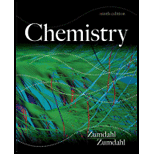# Consider the decomposition equilibrium for dinitrogen pentoxide: 2 N 2 O 5 ( g ) ⇌ 4 NO 2 ( g ) + O 2 ( g ) At a certain temperature and a total pressure of 1.00 atm, the N 2 O 5 is 0.50% decomposed (by moles) at equilibrium. a . If the volume is increased by a factor of 10.0. will the mole percent of N 2 O 5 decomposed at equilibrium be greater than, less than. or equal to 0.50%? Explain your answer. b. Calculate the mole percent of N 2 O 5 that will be decomposed at equilibrium if the volume is increased by a factor of 10.0.### Chemistry

9th Edition
Steven S. Zumdahl
Publisher: Cengage Learning
ISBN: 9781133611097### Chemistry

9th Edition
Steven S. Zumdahl
Publisher: Cengage Learning
ISBN: 9781133611097

#### Solutions

Chapter
Section
Chapter 13, Problem 108CP
Textbook Problem

## Expert Solution

### Want to see the full answer?

Check out a sample textbook solution.See solution

### Want to see this answer and more?

Experts are waiting 24/7 to provide step-by-step solutions in as fast as 30 minutes!*

See Solution

*Response times vary by subject and question complexity. Median response time is 34 minutes and may be longer for new subjects.